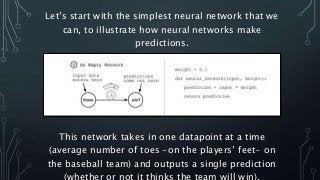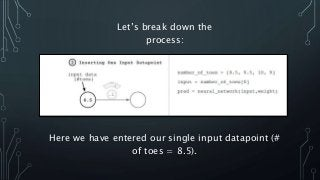Successfully reported this slideshow.

# Grokking Deep Learning: how do neural networks make predictions?×

# Grokking Deep Learning: how do neural networks make predictions?

Artificial Intelligence is one of the most exciting technologies of the century, and Deep Learning is in many ways the "brain" behind some of the world's smartest Artificial Intelligence systems out there.
Grokking Deep Learning is the perfect place to begin your deep learning journey. Rather than just learn the "black box" API of some library or framework, you will actually understand how to build these algorithms completely from scratch.

Save 42% off Grokking Deep Learning with code grokdl at: https://goo.gl/gSSVMK

From manning.com

Artificial Intelligence is one of the most exciting technologies of the century, and Deep Learning is in many ways the "brain" behind some of the world's smartest Artificial Intelligence systems out there.
Grokking Deep Learning is the perfect place to begin your deep learning journey. Rather than just learn the "black box" API of some library or framework, you will actually understand how to build these algorithms completely from scratch.

Save 42% off Grokking Deep Learning with code grokdl at: https://goo.gl/gSSVMK

From manning.com

### Grokking Deep Learning: how do neural networks make predictions?

1. 1. How do neural networks make predictions? Save 42% off Grokking Deep Learning with code grokdl at manning.com
2. 2. Let’s start with the simplest neural network that we can, to illustrate how neural networks make predictions. This network takes in one datapoint at a time (average number of toes -on the players’ feet- on the baseball team) and outputs a single prediction (whether or not it thinks the team will win).
3. 3. Let’s break down the process: Here we have entered our single input datapoint (# of toes = 8.5).
4. 4. Now we’ve got to do a bit of math: Our input is going to be multiplied by the weight (0.1).
5. 5. Out comes the prediction: We’ve shown how a basic neural network predicts something - 8.5 toes x 0.1 = 0.85
6. 6. You might be asking: what exactly is weight in our neural network? Our neural network accepts an input variable as information, and a weight variable as knowledge and outputs a prediction. Every neural network you will ever see works this way. It uses the knowledge in the weights to interpret the information in the input data. Basically, a neural network's weight is a measure of sensitivity between the input of the network and its prediction – like a volume knob.
7. 7. Now let’s see what happens when we have multiple inputs: This example is like our first but it has multiple inputs, rather than just one (# of toes, win/loss, # of fans).
8. 8. We’re going to set our three inputs and corresponding weights: As you can see, the three datapoints have been entered (# of toes, win/loss, # of fans) and the weights set.
9. 9. It’s time for math! We’re going to multiply each of our inputs by our weights (notice that the weights are different) and add them together.
10. 10. Voila! We have our prediction: After filtering our inputs (# of toes, win/loss, # of fans) through our weights (knowledge) we now have a prediction (0.98).
11. 11. That’s pretty cool, right? But, what else can we do with our neural network? Neural Networks can also make multiple predictions using only a single input. This is perhaps a simpler augmentation than multiple inputs. Instead of using more inputs for a single prediction, we are going to make multiple predictions about a single input. The prediction occurs in the same way as if there were 3 disconnected single-weight neural networks – like in the first example.
12. 12. It’s like our first example x 3: This time, we’re going to apply multiple different weights to our input (win/loss, happy/sad, and injuries sustained in %).
13. 13. We insert our datapoints and multiply them with their weights, one at a time: It is important to note that these are, in fact, three separate predictions – unlike our second example, where the multiple inputs were linked to one another.
14. 14. It’s time for math again! Here we are going to perform an elementwise multiplication – like in our first example, but for each weight (0.3, 0.2, and 0.9).
15. 15. We’ve got our 3 predictions! By filtering our input through our various weights (volume knob) we have predicted player injuries, win %, and probability of sadness!
16. 16. What would happen if we combined the methods used in the second and third examples? Yes, neural networks can predict multiple outputs given multiple inputs! The way in which we built a network with multiple inputs or outputs (examples 2 and 3) can be combined together to build a network that has both multiple inputs AND multiple outputs. Just like before, we simply have a weight connecting each input node to each output node and prediction occurs in the usual way.
17. 17. Let’s multiply each of our three inputs by our three weights: We’re going to perform three independent, weighted sums of each input.
18. 18. You can think of this in one of two ways: either as 3 weights coming out of each input node; or 3 weights going into each output node.
19. 19. Here, each output node has taken its own weighted sum of the input and made a prediction.
20. 20. That’s all! Hopefully you found this presentation fun and informative! Save 42% off Grokking Deep Learning with code grokdl at manning.com Check out some of our other deep learning titles: